# Class 8 RD Sharma Solutions – Chapter 20 Area Of Trapezium And Polygon- Exercise 20.2 | Set 2

• Last Updated : 16 Apr, 2021

### Question 11. The cross-section of a canal is a trapezium in shape. If the canal is 10 m wide at the top 6 m wide at the bottom and the area of cross-section is 72 m2 determine its depth.

Solution:

Given:

Length of the parallel sides of the trapezium = 10m and 6m,

Area = 72 m2

Assume that the distance between parallel sides of trapezium is x m

As we know that,

Area of trapezium = 1/2 (Sum of lengths of parallel sides) × distance between parallel sides

Now, put all the given values in this formula, and we get,

72 = 1/2 (10 + 6) × x

72 = 8 × x

x = 72/8 = 9

Hence, the depth is 9m.

### Question 12. The area of a trapezium is 91 cm2 and its height is 7 cm. If one of the parallel sides is longer than the other by 8 cm, find the two parallel sides.

Solution:

Given:

Assume that the length of one parallel side of trapezium = x m,

then, the length of other parallel side of trapezium = (x+8) m,

Area of trapezium = 91 cm2,

Height = 7 cm.

As we know that,

Area of trapezium = 1/2 (Sum of lengths of parallel sides) × altitude

Now, put all the given values in this formula, and we get,

91 = 1/2 (x+x+8) × 7

91 = 1/2(2x+8) × 7

91 = (x+4) × 7

(x+4) = 91/7

x+4 = 13

x = 13 – 4 = 9

Hence, the length of one parallel side of trapezium = 9 cm

Therefore the length of other parallel side of trapezium = x+8 = 9+8 = 17 cm.

### Question 13. The area of a trapezium is 384 cm2. Its parallel sides are in the ratio 3:5 and the perpendicular distance between them is 12 cm. Find the length of each one of the parallel sides.

Solution:

Given:

Assume that the length of one parallel side of trapezium = 3x m,

then the length of other parallel side of trapezium will 5x m,

Area of trapezium = 384 cm2,

Distance between the parallel sides of the trapezium = 12 cm.

As we know that,

Area of trapezium = 1/2 (Sum of lengths of parallel sides) × distance between parallel sides

Now, put all the given values in this formula, and we get,

384 = 1/2 (3x + 5x) × 12

384 = 1/2 (8x) × 12

4x = 384/12

4x = 32

x = 8

Hence, the length of one parallel side of trapezium = 3x = 3× 8 = 24 cm

and length of other parallel side of trapezium = 5x = 5× 8 = 40 cm.

### Question 14. Mohan wants to buy a trapezium shaped field. Its side along the river is parallel and twice the side along the road. If the area of this field is 10500 m2 and the perpendicular distance between the two parallel sides is 100 m, find the length of the side along the river.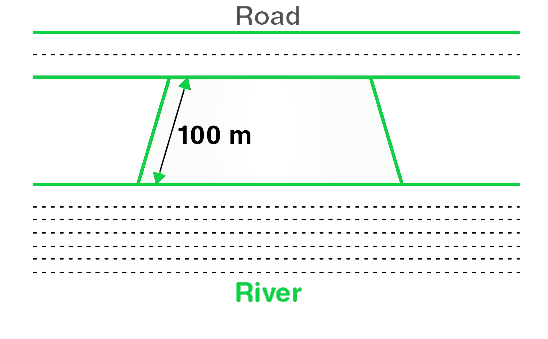Solution:

Given:

Assume that the length of side of trapezium shaped field along road = x m

and length of the other side of trapezium shaped field along road = 2x m,

Area of trapezium = 10500 cm2,

Distance between the parallel sides of the trapezium = 100 m.

As we know that,

Area of trapezium = 1/2 (Sum of lengths of parallel sides) × distance between parallel sides

Now, put all the given values in this formula, and we get,

10500 = 1/2 (x + 2x) × 100

10500 = 1/2 (3x) × 100

3x = 10500/50

3x = 210

x = 210/3 = 70

x = 70

Hence, the length of side of trapezium shaped field along road is 70 m

and length of other side of trapezium shaped field along road will 2x = 70× 2 = 140 m.

### Question 15. The area of a trapezium is 1586 cm2 and the distance between the parallel sides is 26 cm. If one of the parallel sides is 38 cm, find the other.

Solution:

Given:

Let us assume that the length of other parallel side of trapezium = x cm

and Length of one parallel side of trapezium = 38 cm,

Area of trapezium = 1586 cm2,

Distance between parallel sides = 26 cm.

As we know that,

Area of trapezium = 1/2 (Sum of lengths of parallel sides) × distance between parallel sides

Now, put all the given values in this formula, and we get,

1586 = 1/2 (x + 38) × 26

1586 = (x + 38) × 13

(x + 38) = 1586/13

x = 122 – 38

x = 84

Hence, the length of the other parallel side of the trapezium is 84 cm.

### Question 16. The parallel sides of a trapezium are 25 cm and 13 cm; its nonparallel sides are equal, each being 10 cm, find the area of the trapezium.

Solution: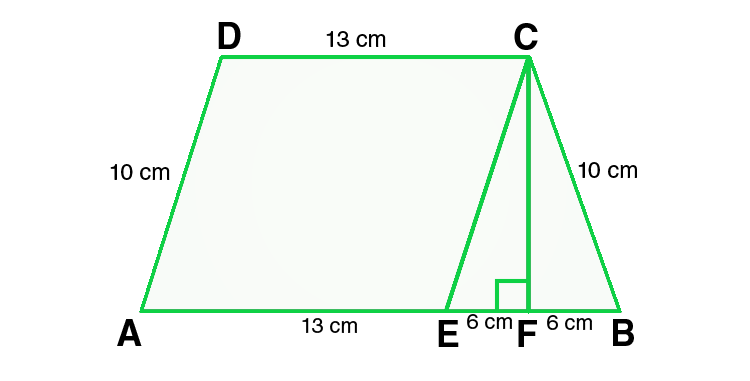Given:

In ΔCEF,

CE = 10 cm and EF = 6cm

By using Pythagoras theorem,

CE2 = CF2 + EF2

CF2 = CE2 – EF2

CF2 = 102 – 62

CF2 = 100 – 36

CF2 = 64

CF = 8 cm

From the figure we conclude that,

Area of trapezium = Area of parallelogram AECD + Area of triangle CEF

= base × height + 1/2 (base × height)

= 13 × 8 + 1/2 (12 × 8) = 104 + 48 = 152

Hence, the area of trapezium is 152 cm2.

### Question 17. Find the area of a trapezium whose parallel sides are 25 cm, 13 cm and the other sides are 15 cm each.

Solution: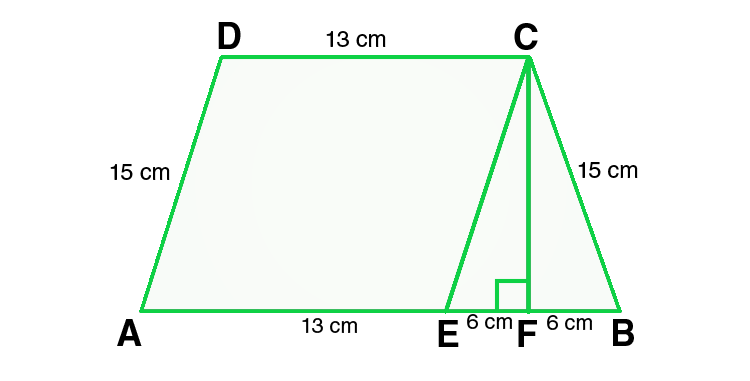Given:

In ΔCEF,

CE = 10 cm and EF = 6cm

By using Pythagoras theorem,

CE2 = CF2 + EF2

CF2 = CE2 – EF2

CF2 = 152 – 62

CF2 = 225 – 36

CF2 = 189

CF = √189 = √ (9×21) = 3√21 cm

From the figure we conclude that,

Area of trapezium = Area of parallelogram AECD + Area of triangle CEF

= height + 1/2 (sum of parallel sides)

= 3√21 × 1/2 (25 + 13)

= 3√21 × 19 = 57√21

Hence, the area of trapezium is 57√21 cm2.

### Question 18. If the area of a trapezium is 28 cm2 and one of its parallel sides is 6 cm, find the other parallel side if its altitude is 4 cm.

Solution:

Given:

Let us assume that the length of other parallel side of trapezium = x cm,

Length of one parallel side of trapezium = 6 cm,

Area of trapezium = 28 cm2,

Length of altitude of trapezium = 4 cm.

As we know that,

Area of trapezium = 1/2 (Sum of lengths of parallel sides) × distance between parallel sides

Now, put all the given values in this formula, and we get,

28 = 1/2 (6 + x) × 4

28 = (6 + x) × 2

(6 + x) = 28/2

(6 + x) = 14

x = 14 – 6 = 8

Hence, the length of the other parallel side of trapezium is 8 cm.

### Question 19. In Fig., a parallelogram is drawn in a trapezium, the area of the parallelogram is 80 cm2, find the area of the trapezium.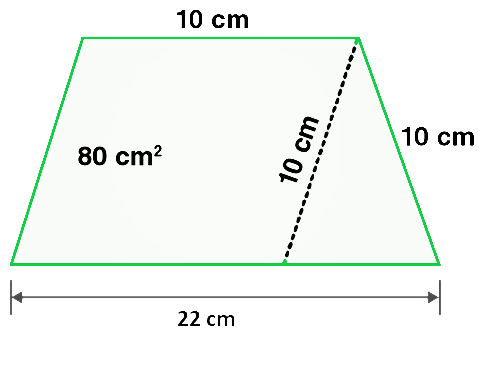Solution: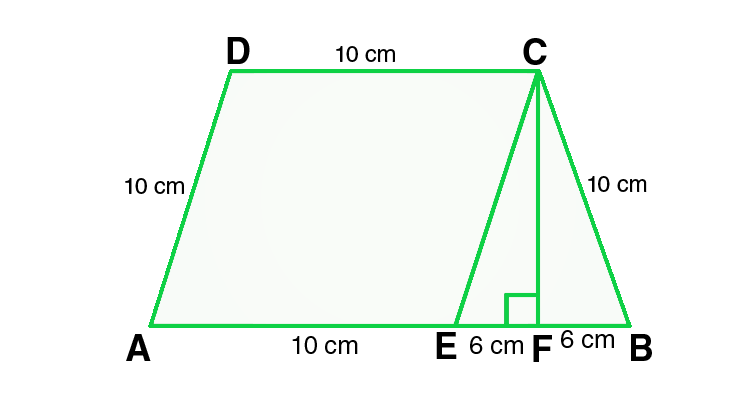Given:

In ΔCEF,

CE = 10 cm and EF = 6cm

By using Pythagoras theorem,

CE2 = CF2 + EF2

CF2 = CE2 – EF2

CF2 = 102 – 62

CF2 = 100-36

CF2 = 64

CF = 8 cm

Area of parallelogram = 80 cm^2            – (Given)

From the figure we conclude that,

Area of trapezium = Area of parallelogram AECD + Area of triangle CEF

So, area of trapezium = base × height + 1/2 (base × height)

Now, put all the given values in this formula, and we get,

= 10 × 8 + 1/2 (12 × 8)

= 80 + 48 = 128

Hence, the area of trapezium is 128 cm2.

### Question 20. Find the area of the field shown in Fig. by dividing it into a square, a rectangle and a trapezium.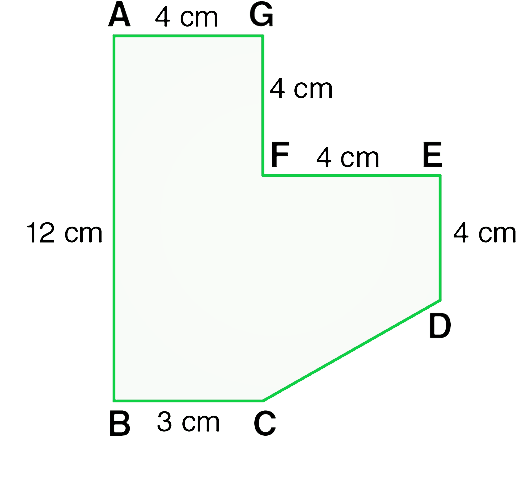Solution: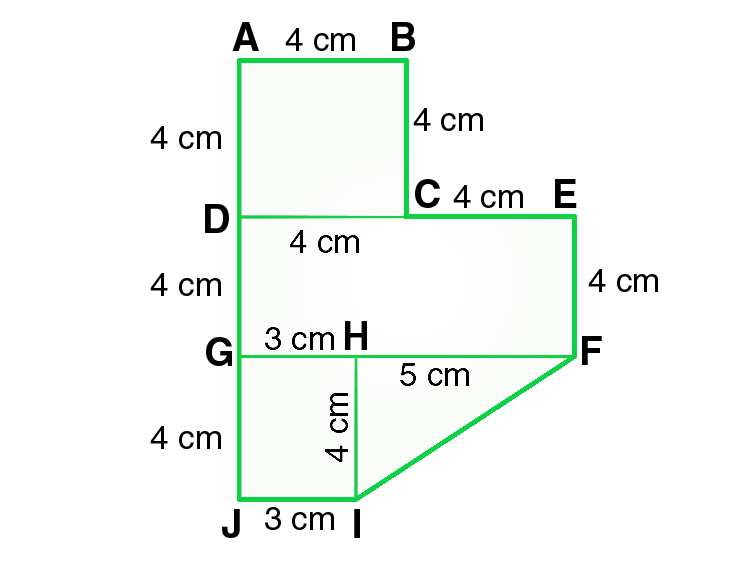From the figure we conclude that,

Area of the given figure = Area of square ABCD + Area of rectangle DEFG +

Area of rectangle GHIJ + Area of triangle FHI

Also,

Area of the given figure = side × side + length × breadth +

length × breadth + 1/2 × base × altitude

Now, put all the given values in this formula, and we get,

= 4×4 + 8×4 + 3×4 + 1/2×5×5

= 16 + 32 + 12 + 10

= 70

Hence, the area of given figure is 70 cm2.

My Personal Notes arrow_drop_up